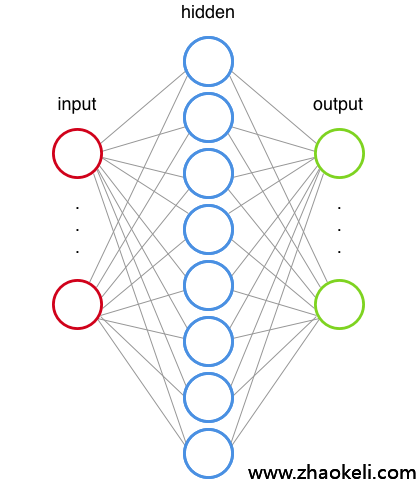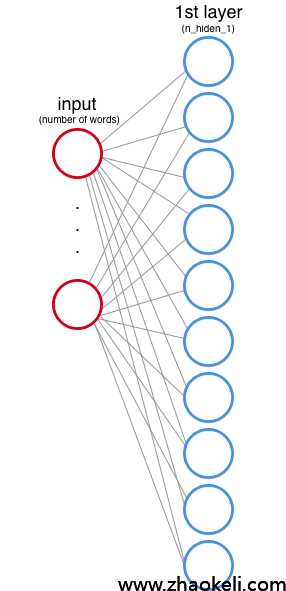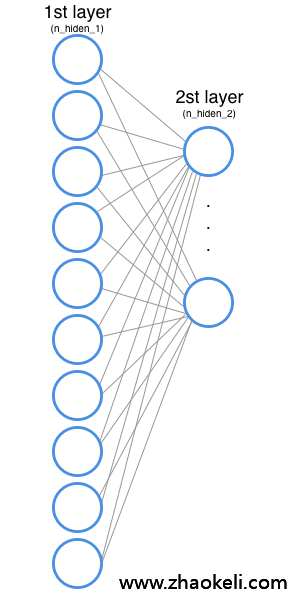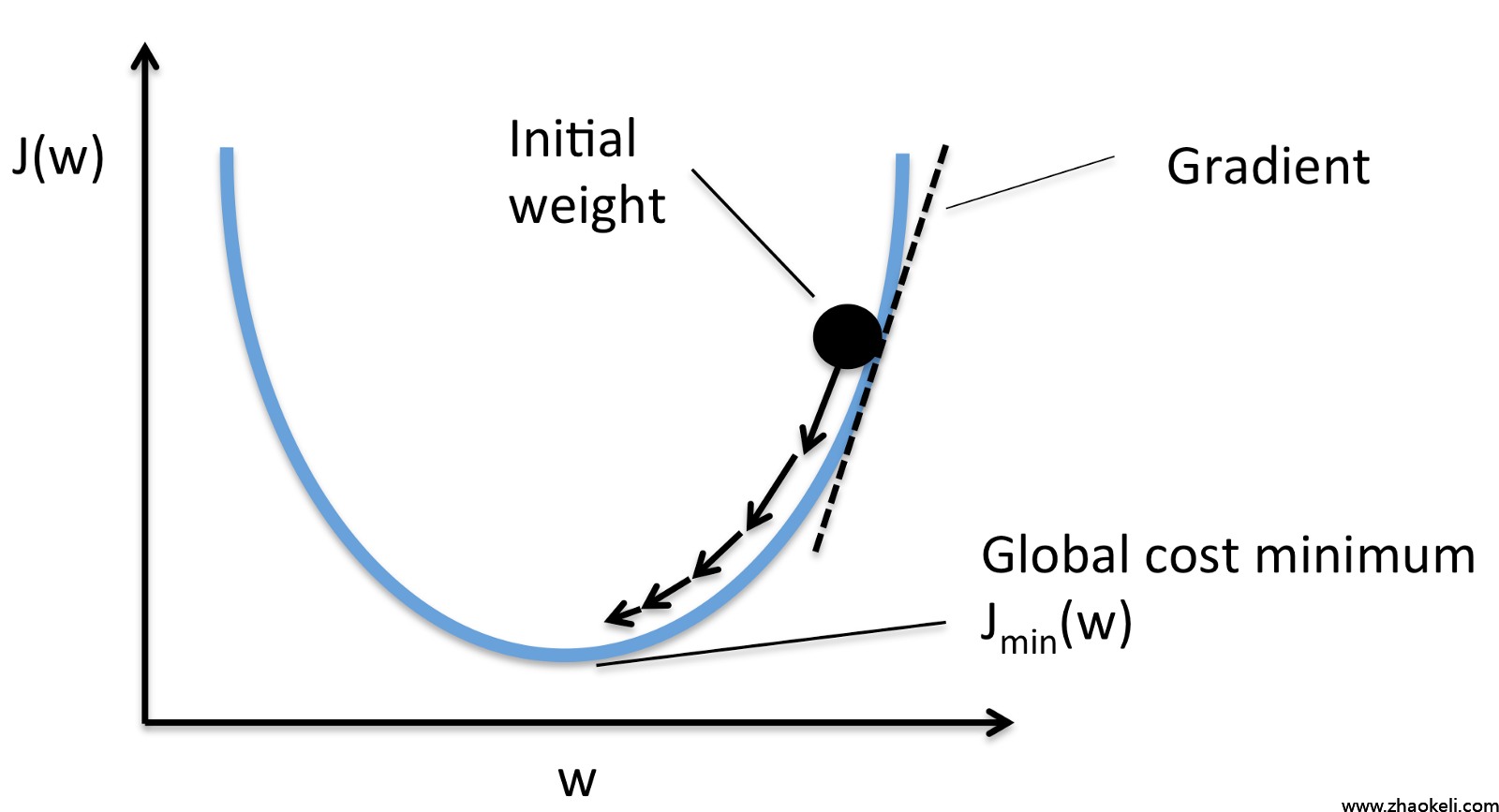# 图解机器学习：神经网络和 TensorFlow 的文本分类 【转】

2019-03-03 15:02:52

TensorFlow 的工作原理

# tf.Graph（计算x+y的图）

```import tensorflow as tf
x = tf.constant([1,3,6])
y = tf.constant([1,1,1])```

```import tensorflow as tf
x = tf.constant([1,3,6])
y = tf.constant([1,1,1])

```import tensorflow as tf
my_graph = tf.Graph()with my_graph.as_default():
x = tf.constant([1,3,6])
y = tf.constant([1,1,1])

# tf.Session

tf.Session 对象封装了 Operation 对象的执行环境。Tensor 对象是被计算过的（从文档中）。为了做到这些，我们需要在 Session 中定义哪个图将被使用到：

```import tensorflow as tf
my_graph = tf.Graph()with tf.Session(graph=my_graph) as sess:
x = tf.constant([1,3,6])
y = tf.constant([1,1,1])

```import tensorflow as tf
my_graph = tf.Graph()with tf.Session(graph=my_graph) as sess:
x = tf.constant([1,3,6])
y = tf.constant([1,1,1])
result = sess.run(fetches=op)
print(result)
>>> [2 4 7]```

# 预测模型（构建预测模型的过程）（预测工作流）

input: text, result: category

“我们知道正确的答案。该算法迭代的预测训练数据，并由老师纠正
” — Jason Brownlee

# 神经网络（一个神经网络）

# 神经网络架构（输入层和第一个隐藏层）

f(x) = max(0,x)  [输出 x 或者 0（零）中最大的数](第一和第二隐藏层)

```+-------------------+-----------+|    category       |   value   |
+-------------------|-----------+|      sports       |    001    |
|      space        |    010    || computer graphics |    100    |
|-------------------|-----------|```

`| 1.2                    0.46|| 0.9   -> [softmax] ->  0.34|| 0.4                    0.20|`

```# Network Parametersn_hidden_1 = 10        # 1st layer number of featuresn_hidden_2 = 5         # 2nd layer number of featuresn_input = total_words  # Words in vocabn_classes = 3          # Categories: graphics, space and baseballdef multilayer_perceptron(input_tensor, weights, biases):
layer_1_multiplication = tf.matmul(input_tensor, weights['h1'])
layer_1_activation = tf.nn.relu(layer_1_addition)# Hidden layer with RELU activation
layer_2_multiplication = tf.matmul(layer_1_activation, weights['h2'])
layer_2_activation = tf.nn.relu(layer_2_addition)# Output layer with linear activation
out_layer_multiplication = tf.matmul(layer_2_activation, weights['out'])

(我们将会在后面讨论输出层的激活函数)

# 神经网络怎么学习

## tf.Variable

```weights = {
'h1': tf.Variable(tf.random_normal([n_input, n_hidden_1])),
'h2': tf.Variable(tf.random_normal([n_hidden_1, n_hidden_2])),
'out': tf.Variable(tf.random_normal([n_hidden_2, n_classes]))
}
biases = {
'b1': tf.Variable(tf.random_normal([n_hidden_1])),
'b2': tf.Variable(tf.random_normal([n_hidden_2])),
'out': tf.Variable(tf.random_normal([n_classes]))
}```

```input values: xweights: w
bias: b
output values: z
expected values: expected```

James D. McCaffrey 写了一个精彩的解释，说明为什么这是这种类型任务的最佳方法。

`# Construct modelprediction = multilayer_perceptron(input_tensor, weights, biases)# Define lossentropy_loss = tf.nn.softmax_cross_entropy_with_logits(logits=prediction, labels=output_tensor)loss = tf.reduce_mean(entropy_loss)`（梯度下降。源: https://sebastianraschka.com/faq/docs/closed-form-vs-gd.html）

`learning_rate = 0.001# Construct modelprediction = multilayer_perceptron(input_tensor, weights, biases)# Define lossentropy_loss = tf.nn.softmax_cross_entropy_with_logits(logits=prediction, labels=output_tensor)loss = tf.reduce_mean(entropy_loss)optimizer = tf.train.AdamOptimizer(learning_rate=learning_rate).minimize(loss)`

# 数据操作

1. 为每一个工作创建索引

2. 为每一个文本创建矩阵，在矩阵里，如果单词在文本中则值为 1，否则值为 0

```import numpy as np    #numpy is a package for scientific computingfrom collections import Counter
vocab = Counter()
text = "Hi from Brazil"#Get all wordsfor word in text.split(' '):    vocab[word]+=1        #Convert words to indexesdef get_word_2_index(vocab):    word2index = {}    for i,word in enumerate(vocab):
word2index[word] = i
return word2index#Now we have an indexword2index = get_word_2_index(vocab)
total_words = len(vocab)#This is how we create a numpy array (our matrix)matrix = np.zeros((total_words),dtype=float)#Now we fill the valuesfor word in text.split():    matrix[word2index[word]] += 1print(matrix)
>>> [ 1.  1.  1.]```

```matrix = np.zeros((total_words),dtype=float)
text = "Hi"for word in text.split():
matrix[word2index[word.lower()]] += 1print(matrix)>>> [ 1.  0.  0.]```

```y = np.zeros((3),dtype=float)if category == 0:
y = 1.        # [ 1.  0.  0.]elif category == 1:
y = 1.        # [ 0.  1.  0.]else:     y = 1.       # [ 0.  0.  1.]```

## 数据集

```from sklearn.datasets import fetch_20newsgroups
categories = ["comp.graphics","sci.space","rec.sport.baseball"]
newsgroups_train = fetch_20newsgroups(subset='train', categories=categories)
newsgroups_test = fetch_20newsgroups(subset='test', categories=categories)```

## 训练模型

feed_dict 参数是我们为每步运行所输入的数据。为了传递这个数据，我们需要定义tf.placeholders（提供给 feed_dict）

“占位符的存在只作为输入的目标，它不需要初始化，也不包含数据。” — Source

```n_input = total_words # Words in vocabn_classes = 3         # Categories: graphics, sci.space and baseballinput_tensor = tf.placeholder(tf.float32,[None, n_input],name="input")
output_tensor = tf.placeholder(tf.float32,[None, n_classes],name="output")```

“如果为了能够输入而使用占位符，可通过使用 tf.placeholder(…, shape=[None, …]) 创建占位符来指定变量批量维度。shape 的 None 元素对应于大小可变的维度。” — Source

get_batches() 函数为我们提供了批处理大小的文本数。现在我们可以运行模型：

```training_epochs = 10# Launch the graphwith tf.Session() as sess:
sess.run(init) #inits the variables (normal distribution, remember?)    # Training cycle    for epoch in range(training_epochs):        avg_cost = 0.        total_batch = int(len(newsgroups_train.data)/batch_size)
# Loop over all batches        for i in range(total_batch):            batch_x,batch_y = get_batch(newsgroups_train,i,batch_size)
# Run optimization op (backprop) and cost op (to get loss value)            c,_ = sess.run([loss,optimizer], feed_dict={input_tensor: batch_x, output_tensor:batch_y})```

```    # Test model    index_prediction = tf.argmax(prediction, 1)
index_correct = tf.argmax(output_tensor, 1)
correct_prediction = tf.equal(index_prediction, index_correct)
# Calculate accuracy    accuracy = tf.reduce_mean(tf.cast(correct_prediction, "float"))
total_test_data = len(newsgroups_test.target)
batch_x_test,batch_y_test = get_batch(newsgroups_test,0,total_test_data)
print("Accuracy:", accuracy.eval({input_tensor: batch_x_test, output_tensor: batch_y_test}))>>> Epoch: 0001 loss= 1133.908114347    Epoch: 0002 loss= 329.093700409    Epoch: 0003 loss= 111.876660109    Epoch: 0004 loss= 72.552971845    Epoch: 0005 loss= 16.673050320    Epoch: 0006 loss= 16.481995190    Epoch: 0007 loss= 4.848220565    Epoch: 0008 loss= 0.759822878    Epoch: 0009 loss= 0.000000000    Epoch: 0010 loss= 0.079848485
Optimization Finished!
Accuracy: 0.75```